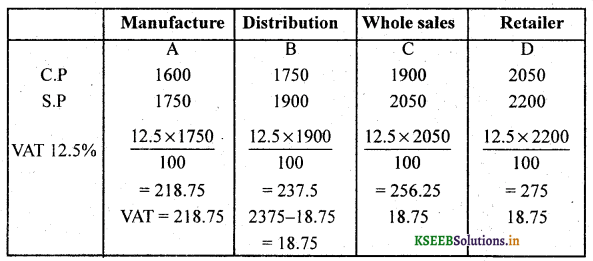# 2nd PUC Basic Maths Question Bank Chapter 12 Sales Tax and Value Added Tax

Students can Download Basic Maths Question Bank Chapter 12 Sales Tax and Value Added Tax Questions and Answers, Notes Pdf, 2nd PUC Basic Maths Question Bank with Answers helps you to revise the complete Karnataka State Board Syllabus and to clear all their doubts, score well in final exams.

## Karnataka 2nd PUC Basic Maths Question Bank Chapter 12 Sales Tax and Value Added Tax

### 2nd PUC Basic Maths Sales Tax and Value Added Tax Two or Three Marks Questions and Answers

Question 1.
Sukhesh paid Rs. 50 as sales tax on a pair of shoes worth Rs. 500. Find the rate of sales tax?
Sales tax percentage = $$\frac{\text { S.T }}{\text { M.P }} \times 100$$
$$=\frac{50}{500} \times 100=10 \%$$

Question 2.
Abhishek Purchase a bicycle costing ₹ 12,000. If the rate of sales tax is 9%. Calculate the total amount payable by him.
Total amount paid = M.P + ST % (M.P)
= 12,000+ 9% of 12,000
= 12,000 + $$\frac{9}{100}$$ x 12,000
= 12,000 + 1080 = 13,080.
= 13,080Question 3.
Ramu paid 60 as sales tax on a Titan Raga watch worth 1200. Find the rate of sales tax.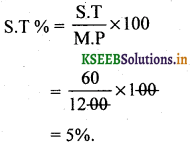Question 4.
Refrigerator is marked for sale for ₹ 17,000 which include sales tax at 10%. Calculate the sales tax in Rs.
S.T = 10% of S.P. Let M.P = x, S.T = ?
Total amount paid (S.P)  = M . P +S.T% of M.P
17,000 = x + 10% (x)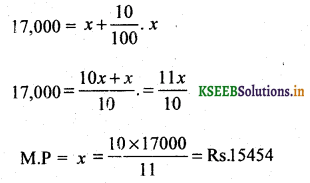∴ S.T = S.P-M.P
= 17,000 – 15454
=1546.

Question 5.
A Furniture dealers sold furniture for ₹ 21,000 and added 5% S.T to the Quoted price. The customer agrees to buy it for ₹ 21,000 including S.T. Find the discount he received. Answer:
Discount = Discount % of P
= 5% of 21,000
$$\frac{5}{100}$$ x 21,000
∴ Customer received discount = ₹ 1050
Amount to be paid = 21,000 + 5% (21,000)                                                 .
= 21,000 + 1050
= 22,050.

Question 6.
A shopkeeper purchases an article for Rs. 9000 and sell it to a customer for Rs. 10500. If the VAT rate is 4%. Find the VAT paid by the shopkeeper.
VAT paid by the shopkeeper
= VAT% of (S.P – CP)
= VAT % of (10,500 – 9000)
= 4% of 1500 = Rs. 60.Question 7.
Sanju a owner of a jewellery shop purchased a ear ring of ₹ 2,000 at 12% VAT and sells it at 2,300 to the customer Radhika. If Radhika also pays 12% VAT to the shopkeeper how much did shopkeeper deposit to the govt as VAT?
C.P2000                                             2300
Vat 12% on 2000                              Vat 12% on 2300
$$\frac{12}{100}$$ x 2,000 = 240            $$\frac{12}{100}$$ x 2300 = 276
∴ VAT charged = 276 – 240 = 36
∴ Total tax paid to government
= 240 + 36
= ₹ 276.

Question 8.
A shopkeeper purchased an electric iron of ₹ 1000 at 8% VAT from the wholesaler and sell it to customer of ₹ 1400 at 8% VAT.
For whole saler Vat = 8% of 1000
= $$=\frac{8 \times 1000}{100}= ₹ 80$$
For buyer Vat = 8% of 1400
= $$\frac{8 \times 1400}{100}$$ = ₹ 112
S.P= 112, C.P = 80
∴ Vat = S.P – C.P
= 112 – 80
= ₹ 32.

Question 9.
Mohan a owner of a departmental store. Purchased an article of 1500 at 4%. VAT and sell it at Rs. 1700 to the customer at 4% VAT. How much amount did the shopkeeper deposit to the governament as VAT.Question 10.
A washing machine is marked for sale for ₹ 17,600 which include sales tax at 10%.
Calculate the sales tax in ₹ ?
S.P = ₹ 17,600, S.T% = 10%, S.T. = ? Let M.P = x
Total amount to be paid (S.P) = M.P + ST% of M.P
17,600 = x + 10% of xS.T = S.P – M.P
= 17,600 – 16,000
= ₹ 1,600.

### 2nd PUC Basic Maths Sales Tax and Value Added Tax Four or Five Marks Questions and Answers

Question 11.
Bharath bought a shirt for ₹ 336, including 12%. Sales tax and a neck tie for ₹ 110 including 10% sales tax. Find the printed price of shirt and neck tie together.
S.P = 336, S.T = 12%, M.P = x
Amount paid = S.P = M.P +12% of M.P∴ The printed price of shirt and neck tie = 300 + 100 = ₹ 400.Question 12.
A shopkeeper sells an item at the price of ₹ 810 including S.T. of 8%. What should a customer pay for the same item. If the S.T is reduced to 6%.
Let the cost price = x
To find cost price =?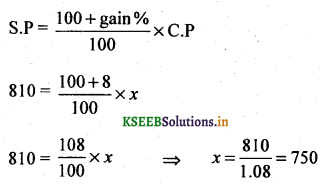∴ Cost price of an item is ₹ 50.
Total amount to be paid = Marked price + S.T% on 750
= 750 + 6% on 750
= 750 + 45 = 795 Rs.
∴ The customer pay for the same item of the S.T is reduced to 6% is ₹ 795.

Question 13.
The price of T.V Set inclusive of sales tax of 9% is ₹ 13,407, find its marked price. If the S.T is increased to 13%. How much more does the customer Sneha pay for the T.V.
Let the M.P be ₹ x
Total amount paid = M.P + S.T% on M.P
13407 = x + 9% (x)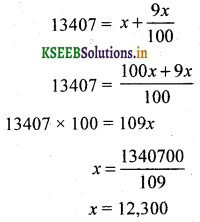x= 12,300
∴ M.P =12,300
Increase in S.T = 13-9 = 4
4% of 12300 = 492 Rs
Marked Price = 12300 + 4% of 12300
= 12300 + 492
= 12792
∴ Rs. 492 should be paid by Sneha.Question 14.
A Shopkeeper bought a TV at a discount of 30% of the listed price of ₹ 24,000. The shopkeeper offer a discount of 10% of the listed price to the customer. If the VAT is 10%. Find (i) The amount paid by the customer (ii) The VAT to be paid by the shopkeeper.
Discount of 10% on 24,000 = 2,400
∴ S.P = 24,000 -2,400 = 21,600
(i) The amount paid by the customer
= 21,600 + 10% of 21,600
= 21,600 + 2160
The amount paid by tire Customer = ₹ 23,760.

(ii) VAT paid by shopkeeper = VAT% (S.P -Shopkeepers S.P)
= 10% (21,600- 16,800)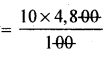= 480

Question 15.
‘A’ is manufacture of Electric Iron. The cost price of each electric iron is 1600. He sells to B and B sells to C and C sells to D to retailer. The tax rate is 12.5% and the profit is – 150 at each stage of the selling chain. Find the (i) The total amount of VAT (ii) The amount that the purchased will have to pay.